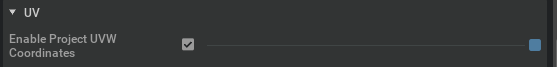# Applying material on terrain mesh from OmnilsaacGymEnvs

Hello,

I am building an extension that generate rough terrain environment in isaac sim. I generate vertices and faces using the source code provided by omniIsaacGymEnvs:

Once the meshes are imported into the stage and can be visualize through the viewport, I want to apply a material texture such as grass for instance. So far I am doing it manually via the GUI but the rendering does not appear. Below is an video example that shows the problem:

You will see that the terrain meshes generated through python api do not change when a texture is applied whereas a plane mesh created form the GUI displays the right texture.
Here is code below that consist of 3 files: terrain_creation.py, terrain_generation_extension.py, terrain_utils.py.
scripts.zip (8.1 KB)

Best,
Yohan

Hi there, thanks for reporting this. I suspect this is because the generated terrain mesh is missing the normals attributes. We will take a further look at this issue internally.

1 Like

I implemented the vertices normals with the following functions:

``````def compute_face_normals(faces, vertices):
n_F = faces.shape
face_normals = np.zeros((n_F, 3))

for i in range(n_F):
a = faces[i, 0]
b = faces[i, 1]
c = faces[i, 2]

A = vertices[a, :]
B = vertices[b, :]
C = vertices[c, :]

BA = B - A
CA = C - A

face_normal = np.cross(BA, CA)

face_normal /= np.linalg.norm(face_normal)

face_normals[i, :] = face_normal

return face_normals
``````
``````def compute_vertex_normals(faces, vertices):
n_V = vertices.shape
vertex_normals = np.zeros((n_V, 3))

# Compute face normals
face_normals = compute_face_normals(vertices, faces)

# Accumulate face normals for each vertex
for i in range(faces.shape):
a = faces[i, 0]
b = faces[i, 1]
c = faces[i, 2]

vertex_normals[a, :] += face_normals[i, :]
vertex_normals[b, :] += face_normals[i, :]
vertex_normals[c, :] += face_normals[i, :]

# Normalize vertex normals
vertex_normals /= np.linalg.norm(vertex_normals, axis=1)[:, np.newaxis]

return vertex_normals
``````

But the meshes still do not show the right texture when a material is assigned to them.

Hi @kellyg - Any news from your side?

Best,
Yohan

Hi @yohan.legars - This will be fixed in the future Isaac Sim release (Expected around June month)

1 Like

Hi there,

The UVW coordinates needed to be enabled.Problem solved !!!

This topic was automatically closed 14 days after the last reply. New replies are no longer allowed.